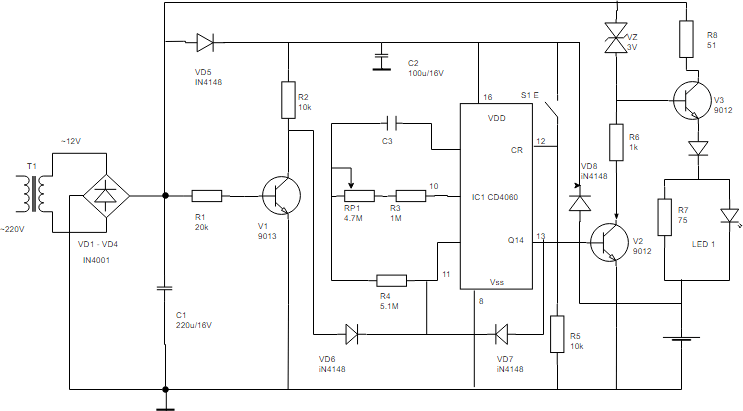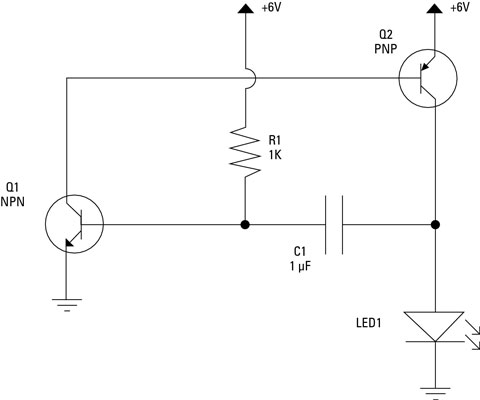# What Is a Basic Schematic Diagram Example?

A schematic diagram is an illustration that shows the electrical connections of a circuit or system. It is typically used to help visualize and understand the functioning of the components within a given system. Schematic diagrams are used in many engineering fields, including electronics, computer science, and mechanical engineering. They can also be used to illustrate the wiring of a complex electronic device or system.

Basic schematic diagrams provide a visual representation of the components in a given system and how they are interconnected. These diagrams allow users to quickly identify various components and their respective functions and connections. Additionally, they provide a visual representation of the flow of electrical current through each component. This is often helpful for troubleshooting and understanding the operation of a system.

## Parts of a Basic Schematic Diagram

A basic schematic diagram usually consists of symbols that represent the different components in the circuit, such as resistors, capacitors, transistors, and other electrical components. These symbols are connected with lines that indicate the electrical connections between each component. The lines may also indicate different voltages, amperage, or other electrical characteristics.

In addition to the symbols, schematic diagrams typically include labels that provide additional information about the components, such as their type and rating. This can be useful for identifying the right parts for a given application. The labels and symbols are usually arranged in a logical order to make it easier to read and interpret the diagram.

## Advantages of Using a Basic Schematic Diagram

Basic schematic diagrams provide a number of advantages over other types of diagrams. For one, they are much easier to read and interpret than other types of diagrams, such as wiring diagrams. This makes them ideal for troubleshooting problems in a system. Additionally, schematic diagrams are easier to update when changes to a system are required.

Another advantage of schematic diagrams is that they can be used to create more complex systems. They provide a visual representation of how components are connected, which makes it easier to design more complex circuits. Additionally, they can be used to construct simulations of a system to test its performance.

Finally, schematic diagrams can also be used to document a system’s components and connections. This can be useful for keeping track of components and making sure they are properly wired.

## Conclusion

Basic schematic diagrams are a useful tool for many engineering disciplines. They provide a visual representation of the components in a system and how they are connected. This makes it easier to understand and troubleshoot the system, as well as to design more complex circuits. Additionally, they can be used to document a system’s components and connections.How To Create Circuit DiagramSchematic Diagram Depicting Examples Of Inputs For Sing The ScientificSchematic Diagram Of Real Impedance Circuit Example The Basic Emc ScientificDifference Between Pictorial And Schematic Diagrams Lucidchart BlogSchematic Diagram Of The Example Communication Network ScientificSchematic Diagram Demonstrating A An Example Of Disordered Ad Hoc ScientificWhat Is A Schematic DiagramWhat Is The Meaning Of Schematic Diagram Sierra CircuitsCircuit Diagram MydrawDifference Between Pictorial And Schematic Diagrams Lucidchart BlogWhat Is The Meaning Of Schematic Diagram Sierra CircuitsCircuit Diagram And Its Components Explanation With SymbolsElectrical Drawings And Schematics OverviewElectronics Schematics Commonly Symbols And Labels Article DummiesSchematic Diagram TemplateSchematic Diagram Of The Electronic Circuit In 22 Inspired By ScientificSchematic Diagram Of The Proportion Respondents Who Were Willing To ScientificCircuit Diagram How To Read And Understand Any Schematic